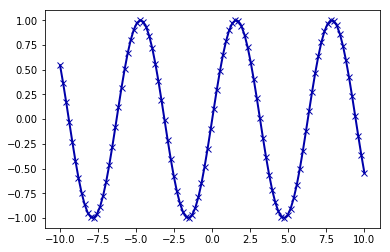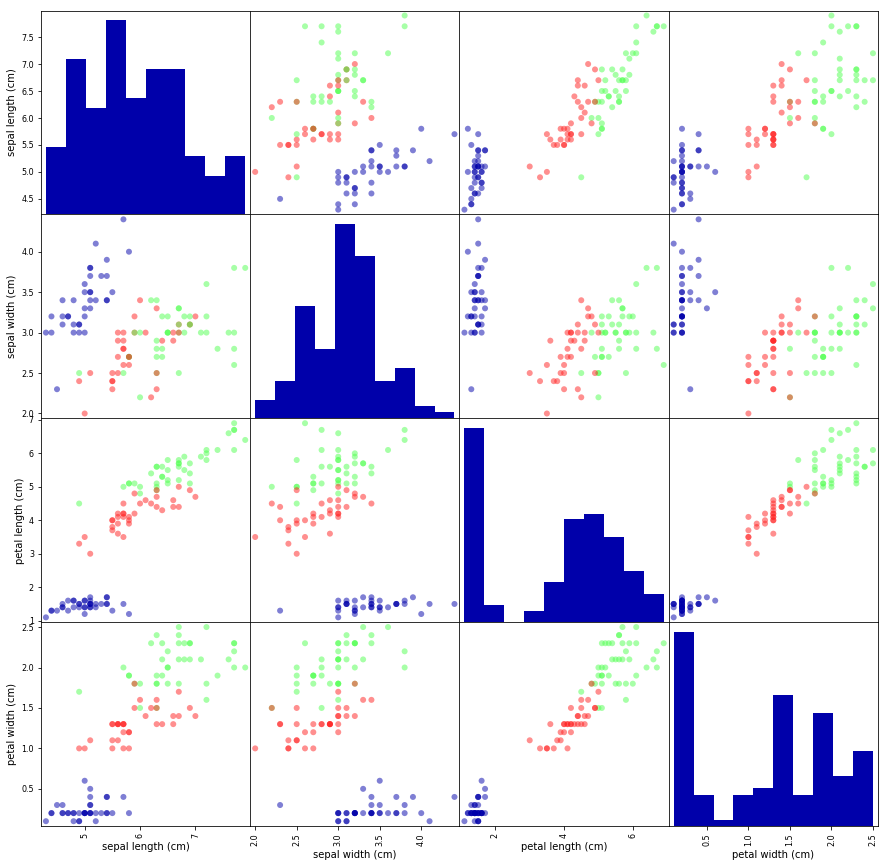In :
from preamble import *
%matplotlib inline


## 1. Introduction¶

### 1.4 Essential Libraries and Tools¶

#### 1.4.2 NumPy¶

In :
import numpy as np

x = np.array([[1, 2, 3], [4, 5, 6]])
print("x:\n{}".format(x))

x:
[[1 2 3]
[4 5 6]]


#### 1.4.3 SciPy¶

In :
from scipy import sparse

# create a 2d NumPy array with a diagonal of ones, and zeros everywhere else
eye = np.eye(4)
print("NumPy array:\n{}".format(eye))

NumPy array:
[[ 1.  0.  0.  0.]
[ 0.  1.  0.  0.]
[ 0.  0.  1.  0.]
[ 0.  0.  0.  1.]]

In :
# convert the NumPy array to a SciPy sparse matrix in CSR (compressed sparse row) format
# only the non-zero entries are stored
sparse_matrix = sparse.csr_matrix(eye)
print("SciPy sparse CSR matrix:\n{}".format(sparse_matrix))

SciPy sparse CSR matrix:
(0, 0)	1.0
(1, 1)	1.0
(2, 2)	1.0
(3, 3)	1.0

In :
data = np.ones(5) * -10
print("data:\n{}".format(data))

row_indices = np.arange(5)
print("row_indices:\n{}".format(row_indices))

col_indices = np.arange(5)
print("col_indices:\n{}".format(col_indices))

# convert the NumPy array to a SciPy sparse matrix in COO (Coordinate) Format
eye_coo = sparse.coo_matrix((data, (row_indices, col_indices)))
print("COO representation:\n{}".format(eye_coo))

data:
[-10. -10. -10. -10. -10.]
row_indices:
[0 1 2 3 4]
col_indices:
[0 1 2 3 4]
COO representation:
(0, 0)	-10.0
(1, 1)	-10.0
(2, 2)	-10.0
(3, 3)	-10.0
(4, 4)	-10.0


#### 1.4.4 matplotlib¶

In :
%matplotlib inline
import matplotlib.pyplot as plt

# Generate a sequence numbers from -10 to 10 with 100 steps in between
x = np.linspace(-10, 10, 100)
print("x:\n{}".format(x))

# create a second array using sinus
y = np.sin(x)
print("y:\n{}".format(y))

# The plot function makes a line chart of one array against another
plt.plot(x, y, marker="x")
plt.show()

x:
[-10.     -9.798  -9.596  -9.394  -9.192  -8.99   -8.788  -8.586  -8.384
-8.182  -7.98   -7.778  -7.576  -7.374  -7.172  -6.97   -6.768  -6.566
-6.364  -6.162  -5.96   -5.758  -5.556  -5.354  -5.152  -4.949  -4.747
-4.545  -4.343  -4.141  -3.939  -3.737  -3.535  -3.333  -3.131  -2.929
-2.727  -2.525  -2.323  -2.121  -1.919  -1.717  -1.515  -1.313  -1.111
-0.909  -0.707  -0.505  -0.303  -0.101   0.101   0.303   0.505   0.707
0.909   1.111   1.313   1.515   1.717   1.919   2.121   2.323   2.525
2.727   2.929   3.131   3.333   3.535   3.737   3.939   4.141   4.343
4.545   4.747   4.949   5.152   5.354   5.556   5.758   5.96    6.162
6.364   6.566   6.768   6.97    7.172   7.374   7.576   7.778   7.98
8.182   8.384   8.586   8.788   8.99    9.192   9.394   9.596   9.798
10.   ]
y:
[ 0.544  0.365  0.17  -0.031 -0.231 -0.421 -0.595 -0.744 -0.863 -0.947
-0.992 -0.997 -0.962 -0.887 -0.776 -0.634 -0.466 -0.279 -0.08   0.121
0.318  0.502  0.665  0.801  0.905  0.972  0.999  0.986  0.933  0.841
0.716  0.561  0.384  0.191 -0.01  -0.211 -0.403 -0.578 -0.73  -0.852
-0.94  -0.989 -0.998 -0.967 -0.896 -0.789 -0.65  -0.484 -0.298 -0.101
0.101  0.298  0.484  0.65   0.789  0.896  0.967  0.998  0.989  0.94
0.852  0.73   0.578  0.403  0.211  0.01  -0.191 -0.384 -0.561 -0.716
-0.841 -0.933 -0.986 -0.999 -0.972 -0.905 -0.801 -0.665 -0.502 -0.318
-0.121  0.08   0.279  0.466  0.634  0.776  0.887  0.962  0.997  0.992
0.947  0.863  0.744  0.595  0.421  0.231  0.031 -0.17  -0.365 -0.544]#### 1.4.5 pandas¶

In :
import pandas as pd
from IPython.display import display

# create a simple dataset of people
data = {'Name': ["John", "Anna", "Peter", "Linda"],
'Location' : ["New York", "Paris", "Berlin", "London"],
'Age' : [24, 13, 53, 33]
}

data_pandas = pd.DataFrame(data)
# IPython.display allows "pretty printing" of dataframes in the Jupyter notebook
display(data_pandas)

# also print it
print(data_pandas)

Age Location Name
0 24 New York John
1 13 Paris Anna
2 53 Berlin Peter
3 33 London Linda
   Age  Location   Name
0   24  New York   John
1   13     Paris   Anna
2   53    Berlin  Peter
3   33    London  Linda

In :
# One of many possible ways to query the table:
# selecting all rows that have an age column greate than 30
display(data_pandas[data_pandas.Age > 30])

Age Location Name
2 53 Berlin Peter
3 33 London Linda

### 1.6 Versions Used in this Book¶

In :
import sys
print("Python version: {}".format(sys.version))

import pandas as pd
print("pandas version: {}".format(pd.__version__))

import matplotlib
print("matplotlib version: {}".format(matplotlib.__version__))

import numpy as np
print("NumPy version: {}".format(np.__version__))

import scipy as sp
print("SciPy version: {}".format(sp.__version__))

import IPython
print("IPython version: {}".format(IPython.__version__))

import sklearn
print("scikit-learn version: {}".format(sklearn.__version__))

Python version: 3.6.3 |Anaconda, Inc.| (default, Oct  6 2017, 12:04:38)
[GCC 4.2.1 Compatible Clang 4.0.1 (tags/RELEASE_401/final)]
pandas version: 0.20.3
matplotlib version: 2.1.0
NumPy version: 1.13.3
SciPy version: 0.19.1
IPython version: 6.1.0
scikit-learn version: 0.19.1


### 1.7 A First Application: Classifying iris species¶#### 1.7.1 Meet the data¶

In :
from sklearn.datasets import load_iris

In :
print("Keys of iris_dataset: {}".format(iris_dataset.keys()))

Keys of iris_dataset: dict_keys(['data', 'target', 'target_names', 'DESCR', 'feature_names'])

In :
print(iris_dataset['DESCR'])

Iris Plants Database
====================

Notes
-----
Data Set Characteristics:
:Number of Instances: 150 (50 in each of three classes)
:Number of Attributes: 4 numeric, predictive attributes and the class
:Attribute Information:
- sepal length in cm
- sepal width in cm
- petal length in cm
- petal width in cm
- class:
- Iris-Setosa
- Iris-Versicolour
- Iris-Virginica
:Summary Statistics:

============== ==== ==== ======= ===== ====================
Min  Max   Mean    SD   Class Correlation
============== ==== ==== ======= ===== ====================
sepal length:   4.3  7.9   5.84   0.83    0.7826
sepal width:    2.0  4.4   3.05   0.43   -0.4194
petal length:   1.0  6.9   3.76   1.76    0.9490  (high!)
petal width:    0.1  2.5   1.20  0.76     0.9565  (high!)
============== ==== ==== ======= ===== ====================

:Missing Attribute Values: None
:Class Distribution: 33.3% for each of 3 classes.
:Creator: R.A. Fisher
:Donor: Michael Marshall (MARSHALL%[email protected])
:Date: July, 1988

This is a copy of UCI ML iris datasets.
http://archive.ics.uci.edu/ml/datasets/Iris

The famous Iris database, first used by Sir R.A Fisher

This is perhaps the best known database to be found in the
pattern recognition literature.  Fisher's paper is a classic in the field and
is referenced frequently to this day.  (See Duda & Hart, for example.)  The
data set contains 3 classes of 50 instances each, where each class refers to a
type of iris plant.  One class is linearly separable from the other 2; the
latter are NOT linearly separable from each other.

References
----------
- Fisher,R.A. "The use of multiple measurements in taxonomic problems"
Annual Eugenics, 7, Part II, 179-188 (1936); also in "Contributions to
Mathematical Statistics" (John Wiley, NY, 1950).
- Duda,R.O., & Hart,P.E. (1973) Pattern Classification and Scene Analysis.
(Q327.D83) John Wiley & Sons.  ISBN 0-471-22361-1.  See page 218.
- Dasarathy, B.V. (1980) "Nosing Around the Neighborhood: A New System
Structure and Classification Rule for Recognition in Partially Exposed
Environments".  IEEE Transactions on Pattern Analysis and Machine
Intelligence, Vol. PAMI-2, No. 1, 67-71.
- Gates, G.W. (1972) "The Reduced Nearest Neighbor Rule".  IEEE Transactions
on Information Theory, May 1972, 431-433.
- See also: 1988 MLC Proceedings, 54-64.  Cheeseman et al"s AUTOCLASS II
conceptual clustering system finds 3 classes in the data.
- Many, many more ...


In :
print("Target names: {}".format(iris_dataset['target_names']))

Target names: ['setosa' 'versicolor' 'virginica']

In :
print("Feature names: {}".format(iris_dataset['feature_names']))

Feature names: ['sepal length (cm)', 'sepal width (cm)', 'petal length (cm)', 'petal width (cm)']

In :
print("Type of data: {}".format(type(iris_dataset['data'])))

Type of data: <class 'numpy.ndarray'>

In :
print("Shape of data: {}".format(iris_dataset['data'].shape))

Shape of data: (150, 4)

In :
print("First five rows of data:\n{}".format(iris_dataset['data'][:5]))

First five rows of data:
[[ 5.1  3.5  1.4  0.2]
[ 4.9  3.   1.4  0.2]
[ 4.7  3.2  1.3  0.2]
[ 4.6  3.1  1.5  0.2]
[ 5.   3.6  1.4  0.2]]

In :
print("Type of target: {}".format(type(iris_dataset['target'])))

Type of target: <class 'numpy.ndarray'>

In :
print("Shape of target: {}".format(iris_dataset['target'].shape))

Shape of target: (150,)

In :
print("Target:\n{}".format(iris_dataset['target']))

Target:
[0 0 0 0 0 0 0 0 0 0 0 0 0 0 0 0 0 0 0 0 0 0 0 0 0 0 0 0 0 0 0 0 0 0 0 0 0
0 0 0 0 0 0 0 0 0 0 0 0 0 1 1 1 1 1 1 1 1 1 1 1 1 1 1 1 1 1 1 1 1 1 1 1 1
1 1 1 1 1 1 1 1 1 1 1 1 1 1 1 1 1 1 1 1 1 1 1 1 1 1 2 2 2 2 2 2 2 2 2 2 2
2 2 2 2 2 2 2 2 2 2 2 2 2 2 2 2 2 2 2 2 2 2 2 2 2 2 2 2 2 2 2 2 2 2 2 2 2
2 2]


#### 1.7.2 Measuring Success: Training and testing data¶

In :
from sklearn.model_selection import train_test_split

X_train, X_test, y_train, y_test = train_test_split(
iris_dataset['data'],
iris_dataset['target'],
#test_size=38,
random_state=0
)

In :
print("X_train shape: {}".format(X_train.shape))
print("y_train shape: {}".format(y_train.shape))

X_train shape: (112, 4)
y_train shape: (112,)

In :
print("X_test shape: {}".format(X_test.shape))
print("y_test shape: {}".format(y_test.shape))

X_test shape: (38, 4)
y_test shape: (38,)


#### 1.7.3 First things first: Look at your data¶

In :
# create dataframe from data in X_train
# label the columns using the strings in iris_dataset.feature_names
iris_dataframe = pd.DataFrame(X_train, columns=iris_dataset.feature_names)

# create a scatter matrix from the dataframe, color by y_train
pd.plotting.scatter_matrix(
iris_dataframe,
c=y_train,
figsize=(15, 15),
marker='o',
cmap=mglearn.cm3
)

Out:
array([[<matplotlib.axes._subplots.AxesSubplot object at 0x112458e48>,
<matplotlib.axes._subplots.AxesSubplot object at 0x1145e1780>,
<matplotlib.axes._subplots.AxesSubplot object at 0x11862dcc0>,
<matplotlib.axes._subplots.AxesSubplot object at 0x1185aaef0>],
[<matplotlib.axes._subplots.AxesSubplot object at 0x1185dfef0>,
<matplotlib.axes._subplots.AxesSubplot object at 0x1185dff28>,
<matplotlib.axes._subplots.AxesSubplot object at 0x118702cc0>,
<matplotlib.axes._subplots.AxesSubplot object at 0x11873ab70>],
[<matplotlib.axes._subplots.AxesSubplot object at 0x11875c898>,
<matplotlib.axes._subplots.AxesSubplot object at 0x1186d0e80>,
<matplotlib.axes._subplots.AxesSubplot object at 0x1187d9fd0>,
<matplotlib.axes._subplots.AxesSubplot object at 0x118814fd0>],
[<matplotlib.axes._subplots.AxesSubplot object at 0x118850f60>,
<matplotlib.axes._subplots.AxesSubplot object at 0x118886ef0>,
<matplotlib.axes._subplots.AxesSubplot object at 0x1188bb940>,
<matplotlib.axes._subplots.AxesSubplot object at 0x1188f6da0>]], dtype=object)#### 1.7.4 Building your first model: k nearest neighbors¶

In :
from sklearn.neighbors import KNeighborsClassifier
knn = KNeighborsClassifier(n_neighbors=1)

In :
knn.fit(X_train, y_train)

Out:
KNeighborsClassifier(algorithm='auto', leaf_size=30, metric='minkowski',
metric_params=None, n_jobs=1, n_neighbors=1, p=2,
weights='uniform')

#### 1.7.5 Making predictions¶

In :
X_new = np.array([[5, 2.9, 1, 0.2]])
print("X_new.shape: {}".format(X_new.shape))

X_new.shape: (1, 4)

In :
prediction = knn.predict(X_new)
print("Prediction: {}".format(prediction))
print("Predicted target name: {}".format(
iris_dataset['target_names'][prediction]
))

Prediction: 
Predicted target name: ['setosa']


#### 1.7.6 Evaluating the model¶

In :
y_pred = knn.predict(X_test)
print("Test set predictions:\n {}".format(y_pred))

Test set predictions:
[2 1 0 2 0 2 0 1 1 1 2 1 1 1 1 0 1 1 0 0 2 1 0 0 2 0 0 1 1 0 2 1 0 2 2 1 0
2]

In :
print("Test set score: {:.2f}".format(np.mean(y_pred == y_test)))

Test set score: 0.97

In :
print("Test set score: {:.2f}".format(knn.score(X_test, y_test)))

Test set score: 0.97


### 1.8 Summary and Outlook¶

In :
X_train, X_test, y_train, y_test = train_test_split(
iris_dataset['data'], iris_dataset['target'], random_state=0
)

knn = KNeighborsClassifier(n_neighbors=1)

knn.fit(X_train, y_train)

print("Test set score: {:.2f}".format(knn.score(X_test, y_test)))

Test set score: 0.97